• 微分流形数学书非欧几何书微分流形数学书非欧几何书微分流形数学书非欧几何书
• 本文主要是微分流形课（一般是指第一次上的微分流形课）的计算公式一览。因为不同的体系和作者，使用的记号和叙述可能不一样（比如联络那里），所以这里特别强调课本对应陈省身先生的《微分几何初步》。以下是pdf...
本文主要是微分流形课（一般是指第一次上的微分流形课）的计算公式一览。因为不同的体系和作者，使用的记号和叙述可能不一样（比如联络那里），所以这里特别强调课本对应陈省身先生的《微分几何初步》。以下是pdf截图，文末附latex的tex文件。因为对于初学者，可能有同学会进入一种“看了，懂了，原来如此，但是计算一做就废”的感觉，所以笔者整理了一下公式。笔者整理时难免有错漏，欢迎指正多多包涵。内容主要涉及从张量开始到Gauss-Bonnet公式。并且部分公式后面还有证明简述。（下面为pdf截图）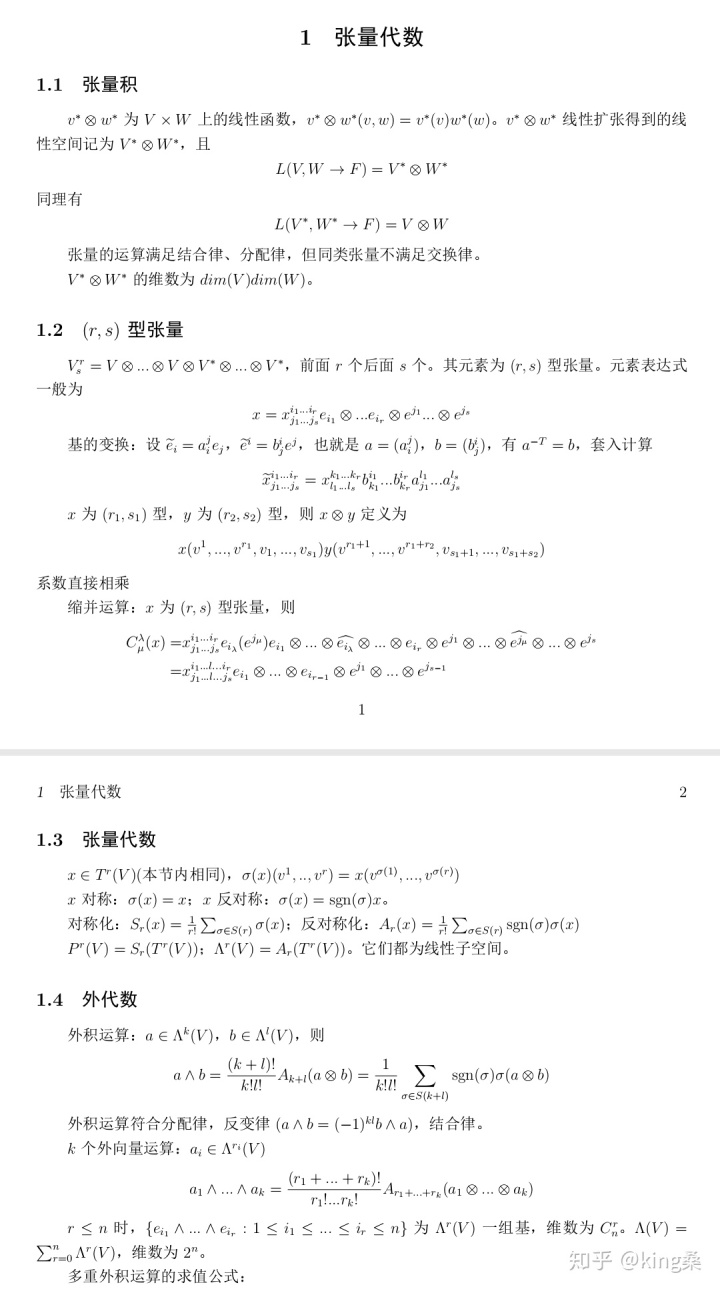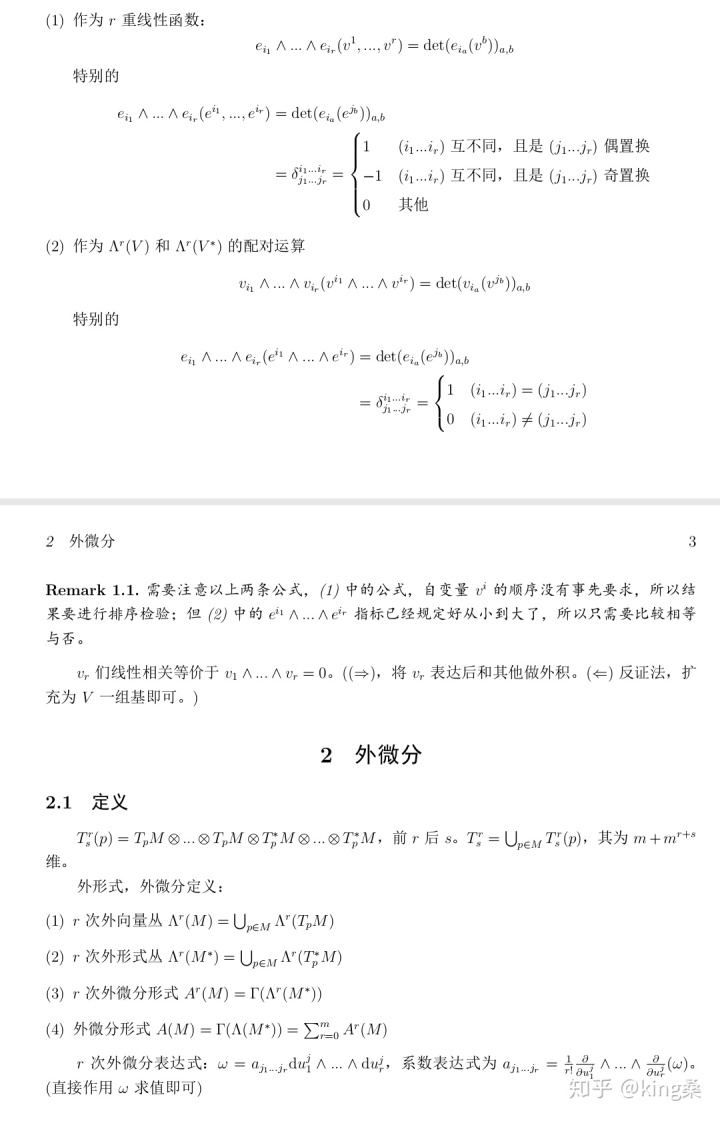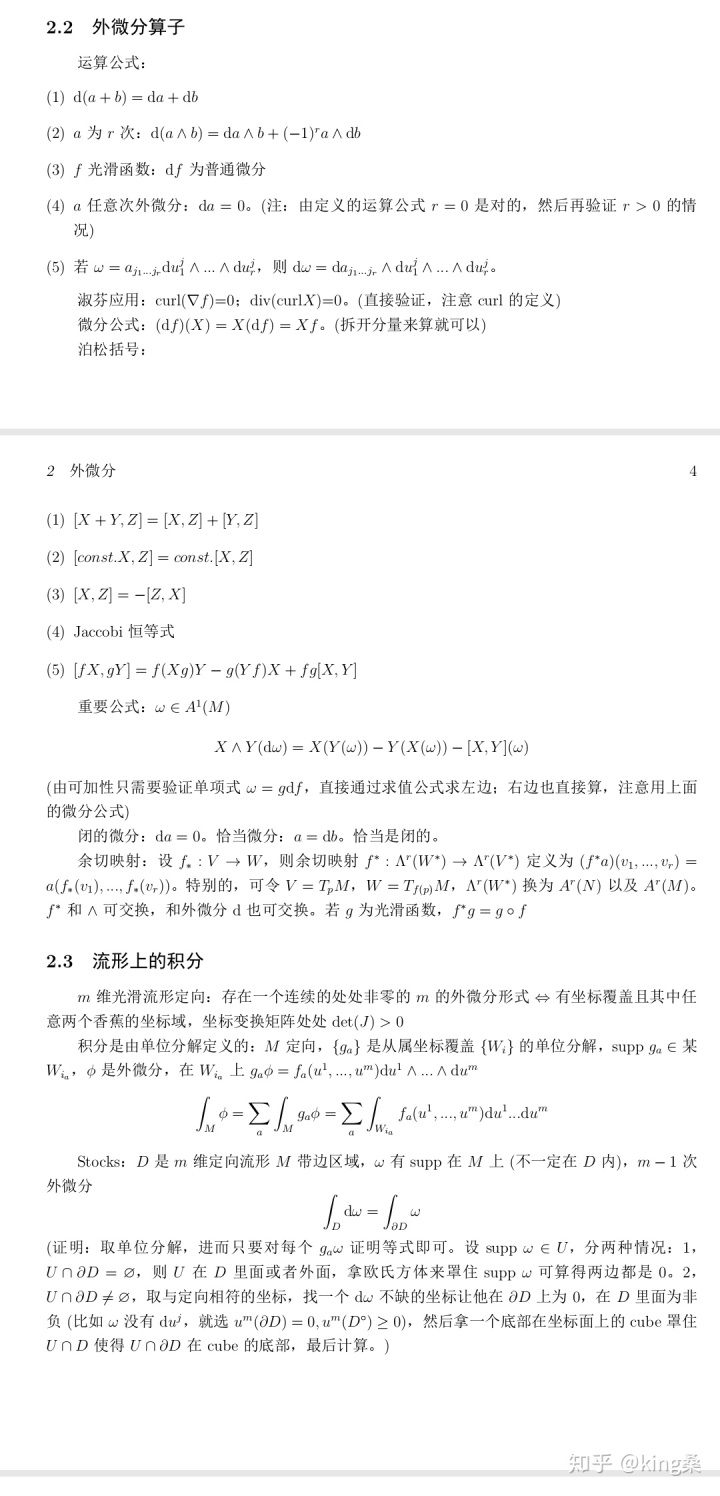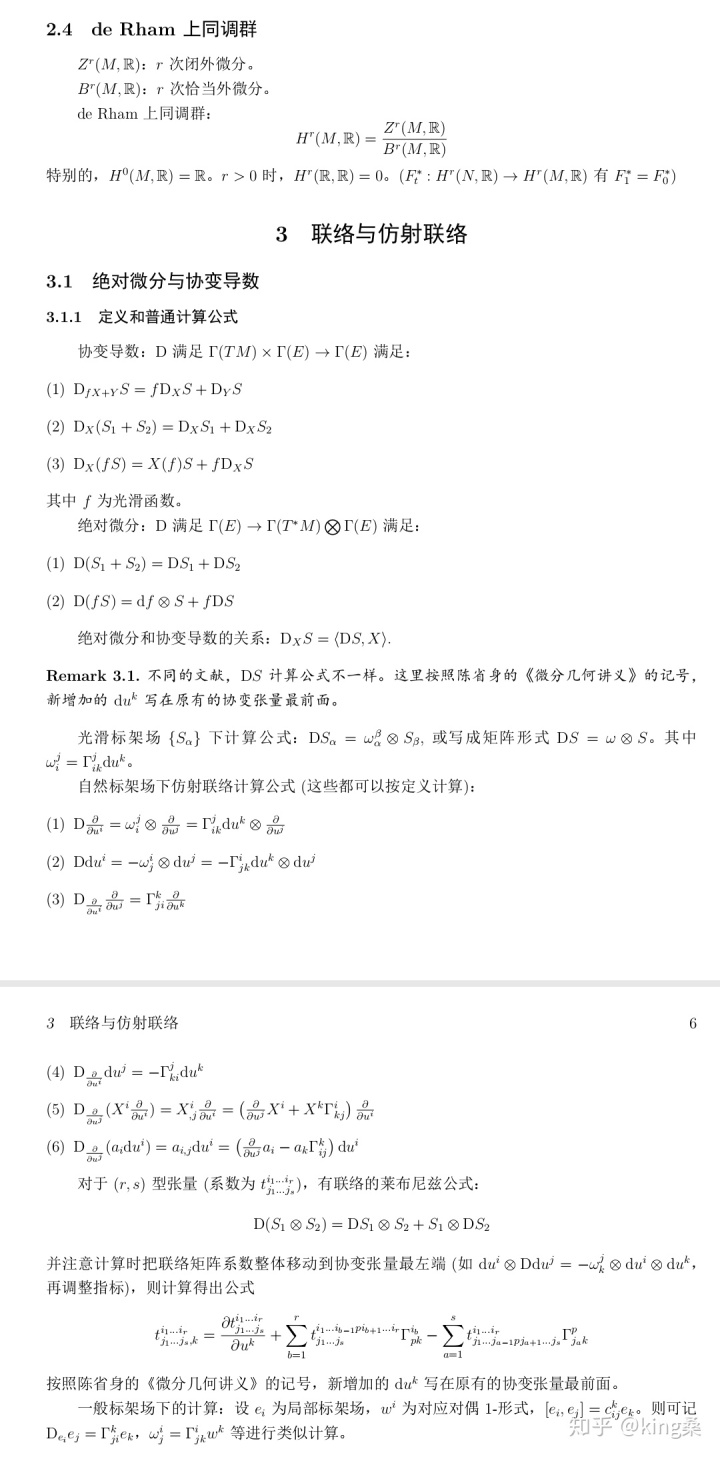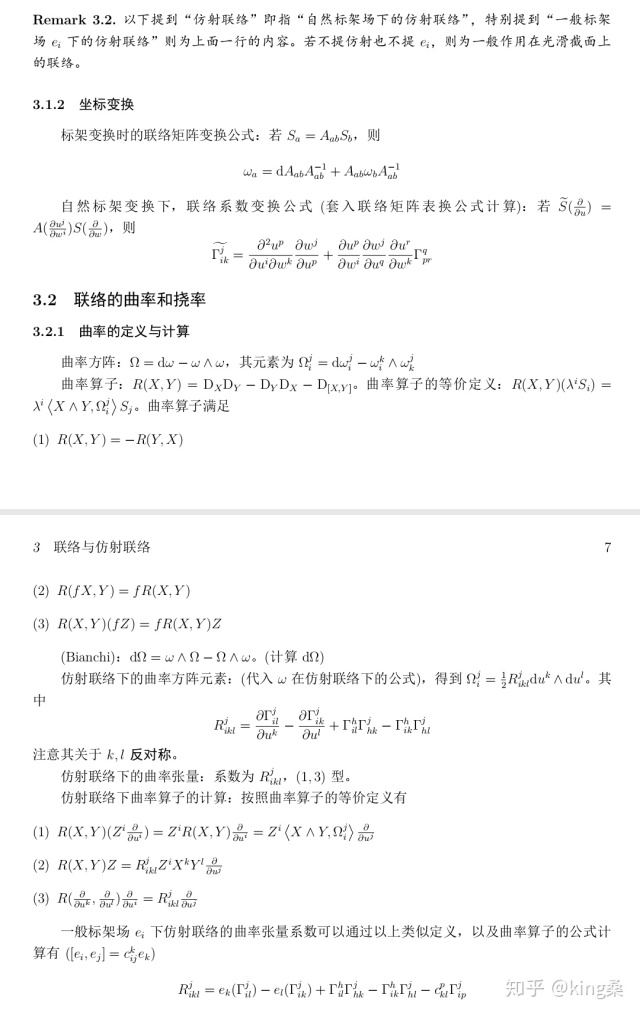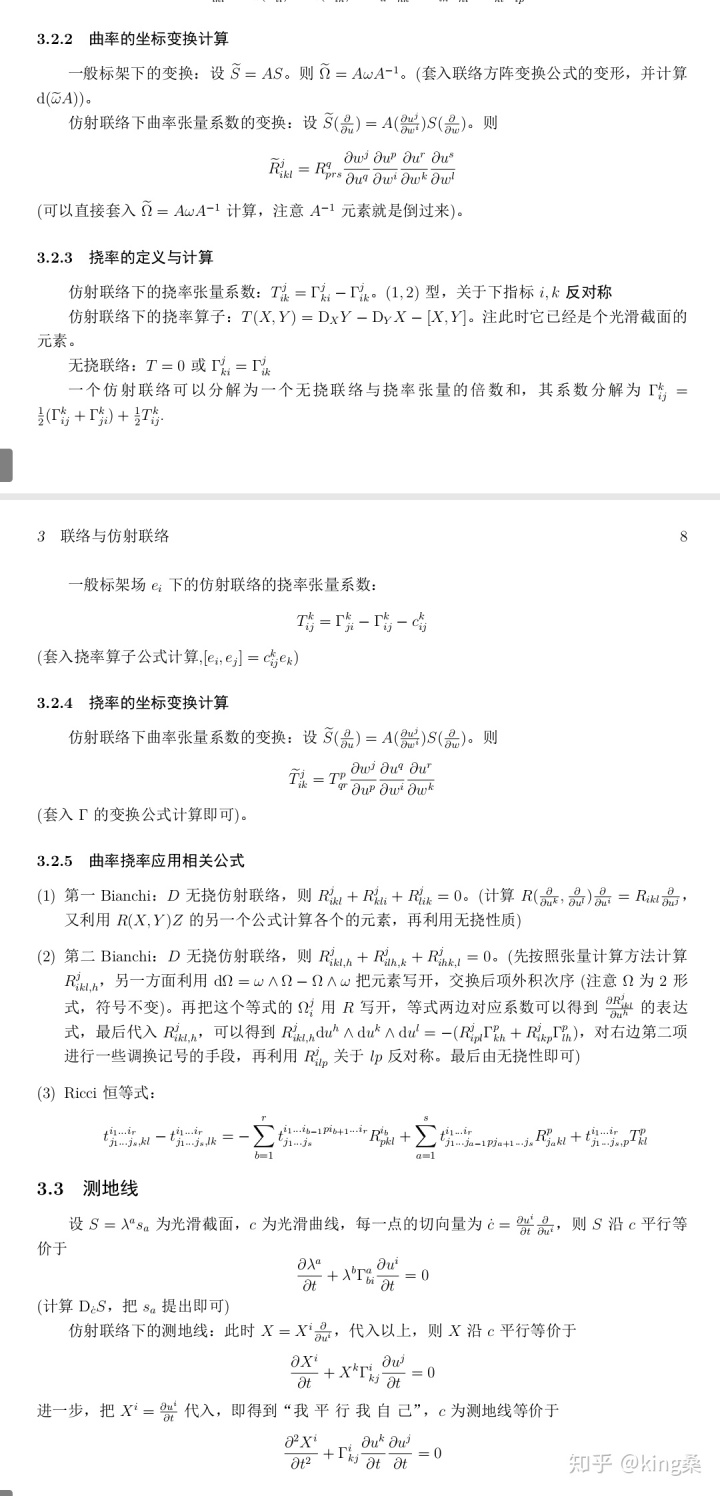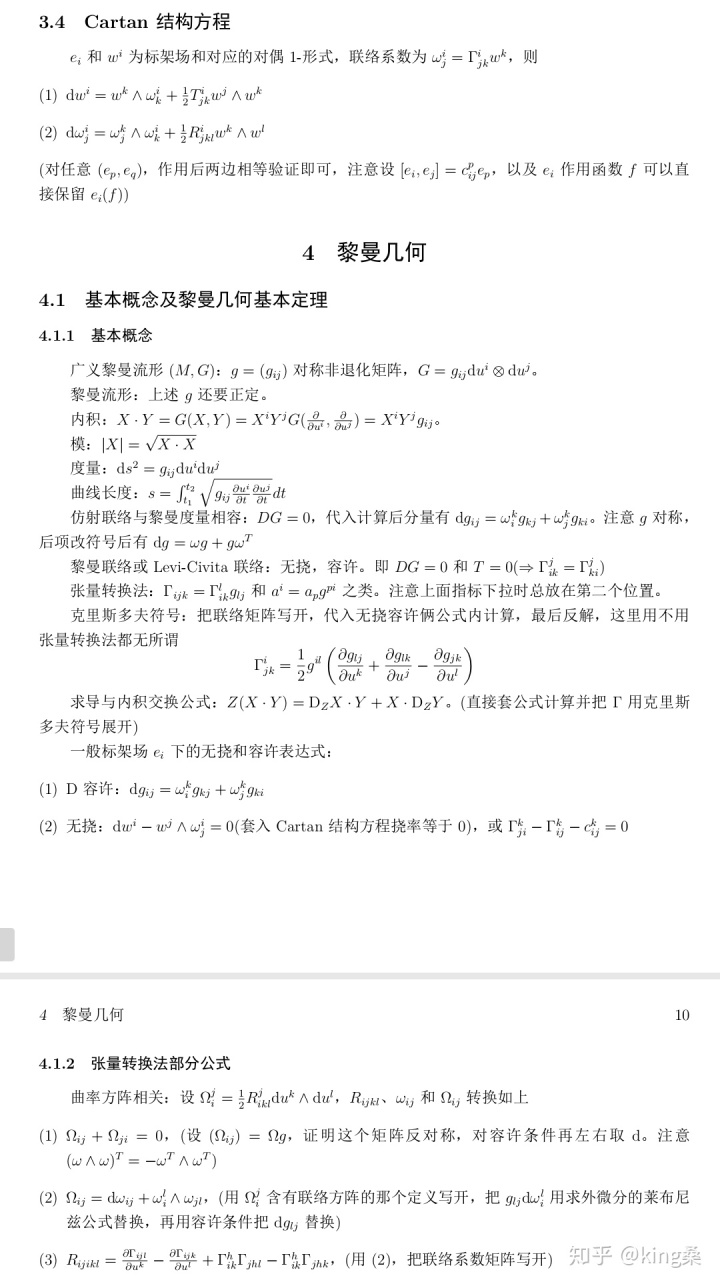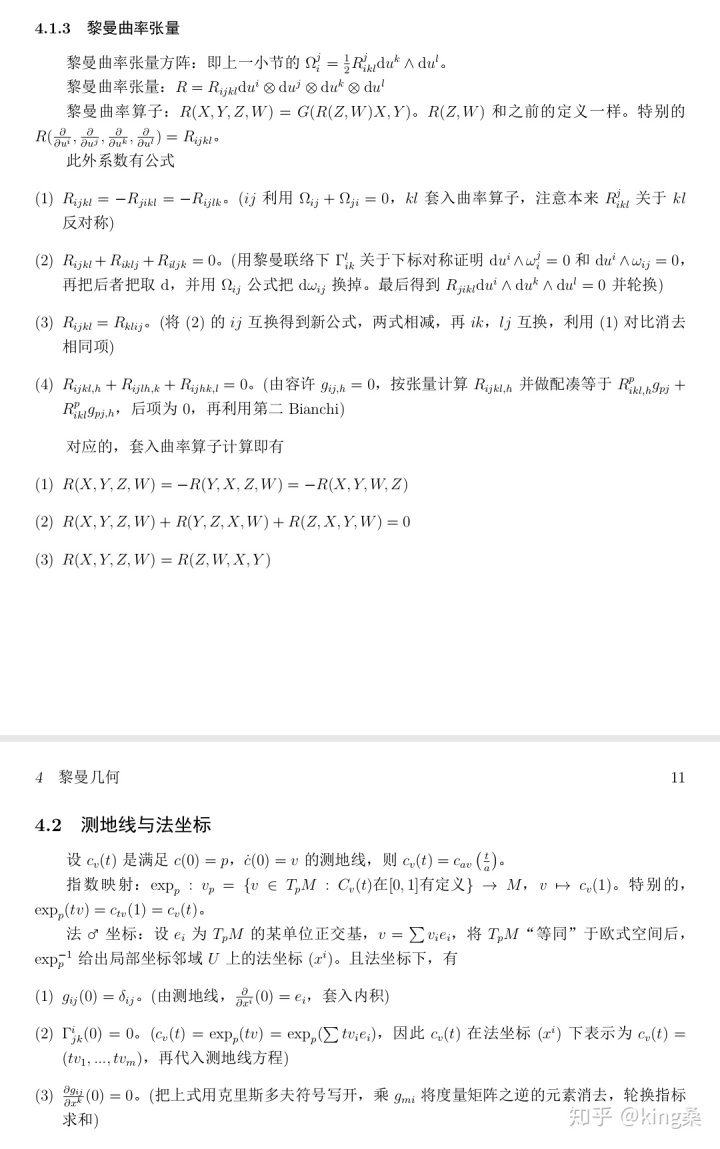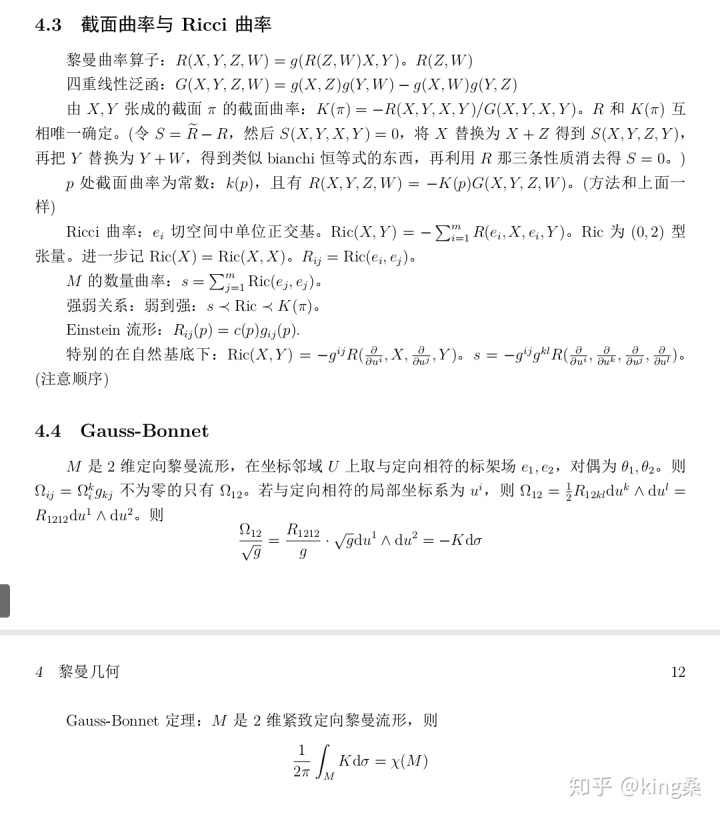tex文件：由于zh管理员硬是认为学习资料是辣鸡广告链接 所以我没法放了 要的私信一下吧（不再补充更深入的知识点了咕咕咕，更深入知识点请各位找书继续学习吧
展开全文•研究论文
• 微分流形初步(陈维桓)(第二版) 微分流形初步(陈维桓)(第二版)
• 我们知道[公式]中存在一个整体的坐标...刚接触微分流形的概念理解起来还是比较困难，因此找来一些具体的例子，可以帮助大家理解微分流形的概念。 微分流形 例１： nnn维球面Sn={(x1,…,xn+1)∈Rn+1∣∑i=1n+1xi2=1}\ma.我们知道[公式]中存在一个整体的坐标系，使得每个点都有一个坐标与之一一对应，大学之前学习的几何内容，都是在[公式]空间中处理问题。但是存在一些空间，在其上不存在一个整体的坐标系，如三维空间中的球面：[公式]。要想在其上处理问题，就需要将其分为若干个部分，在每个部分上建立坐标系，这就引出了微分流形的概念。
刚接触微分流形的概念理解起来还是比较困难，因此找来一些具体的例子，可以帮助大家理解微分流形的概念。
微分流形
例１： $n$维球面$\mathrm{S}^n=\{(x_1,\dots,x_{n+1})\in \mathrm{R}^{n+1}|\sum\limits_{i=1}^{n+1}x_i^2=1\}$是$n$维$C^\infty$微分流形。
证明： 取$\mathrm{S}^n$的拓扑为其作为$\mathrm{R}^{n+1}$子空间的拓扑，则$\mathrm{S}^n$是Hausdorff拓扑空间。令
$U_i^+=\{(x_1,\dots,x_{n+1})\in\mathrm{S}^n|x_i>0\}\\ U_i^-=\{(x_1,\dots,x_{n+1})\in\mathrm{S}^n|x_i<0\}$
$\varphi_i^+:U_i^+\to \mathrm{R}^n,\ (x_1,\dots,x_{n+1})\mapsto (x_1,\dots,\hat{x_i},\dots,x_{n+1})\\ \varphi_i^-:U_i^-\to \mathrm{R}^n,\ (x_1,\dots,x_{n+1})\mapsto (x_1,\dots,\hat{x_i},\dots,x_{n+1})$
其中$i=1,2,\dots,n+1$，则$\varphi_i^+$与$\varphi_i^-$都是可逆映射，有
$(\varphi_i^+)^{-1}:\varphi(U_i^+)\to U_i^+,\ (x_1,\dots,x_n)\mapsto (x_1,\dots,x_{i-1},\sqrt{1-\sum\limits_{j=1}^nx_j^2},x_{i},\dots,x_n)\\ (\varphi_i^-)^{-1}:\varphi(U_i^-)\to U_i^-,\ (x_1,\dots,x_n)\mapsto (x_1,\dots,x_{i-1},-\sqrt{1-\sum\limits_{j=1}^nx_j^2},x_{i},\dots,x_n).$
考虑映射
$\varphi_2^-(\varphi_1^+)^{-1}:\varphi_1^+(U_1^+\cap U_2^-)\to\varphi_2^-(U_1^+\cap U_2^-)\\ (x_1,\dots,x_n)\mapsto (\sqrt{1-\sum\limits_{j=1}^nx_j^2},x_2,\dots,x_n),$
可知$\varphi_2^-(\varphi_1^+)^{-1}$是$C^\infty$映射，因此坐标图$(U_1^+,\varphi_1^+)$与$(U_2^-,\varphi_2^-)$是$C^\infty$相容的。同理可得坐标图册$\{(U_i^\pm,\varphi_i^\pm)|i=1,2,\dots,n+1\}$是$C^\infty$相容坐标图册，因此唯一确定$\mathrm{S}^n$上的$C^\infty$微分结构，因此$\mathrm{S}^n$是$n$维$C^\infty$微分流形。
例２： 实射影空间$\mathrm{R}\mathrm{P}^n$是$n$维$C^\infty$微分流形。
证明： 实射影空间$\mathrm{R}\mathrm{P}^n$是Hausdorff空间。令$X=\mathrm{R}^{n+1}-{0}$，在$X$上定义等价关系$\sim$：对$\forall x,y\in X$，$x\sim y$当且仅当存在$t\in\mathrm{R}$且$t>0$使得$y=tx$，则$\mathrm{R}\mathrm{P}^n$即为$X/\sim$.令
$U_i=\{[(x_1,\dots,x_{n+1})]|(x_1,\dots,x_{n+1})\in X|x_i\ne 0\},\ i=1,2,\dots,n+1$
其中$[x]$表示$x\in X$关于等价关系$\sim$的等价类。令$(x_1,\dots,x_{n+1})\in X$且$x_i\ne 0$，对$\forall (y_1,\dots,y_{n+1})\in X$，可知
$(\frac{x_1}{x_i},\dots,\frac{x_{n+1}}{x_i})=(\frac{y_1}{y_i},\dots,\frac{y_{n+1}}{y_i})\iff(x_1,\dots,x_{n+1})\sim(y_1,\dots,y_{n+1})$
因此，下面的映射$\varphi_i$是良定义的单射
$\varphi_i:U_i\to \mathrm{R}^n,\ [(x_1,\dots,x_{n+1})]\mapsto (\frac{x_1}{x_i},\dots,\frac{x_{i-1}}{x_i},\frac{x_{i+1}}{x_i},\dots,\frac{x_{n+1}}{x_i}),\ i=1,2,\dots,n+1.$
因此$\varphi_i$存在逆映射
$\varphi_i^{-1}:\varphi_i(U_i)\to U_i,\ (x_1,\dots,x_n)\mapsto [(x_1,\dots,x_{i-1},1,x_{i+1},\dots,x_{n+1})].$
考虑映射
$\varphi_2(\varphi_1)^{-1}:\varphi_1(U_1\cap U_2)\to \varphi_2(U_1\cap U_2)\\ (x_1,\dots,x_n)\mapsto (\frac{1}{x_1},\frac{x_2}{x_1},\dots,\frac{x_{n}}{x_1}),$
可知$\varphi_2(\varphi_1)^{-1}$是$C^\infty$映射，因此坐标图$(U_1,\varphi_1)$与$(U_2,\varphi_2)$是$C^\infty$相容的。同理可得坐标图册$\{(U_i,\varphi_i)|i=1,2,\dots,n+1\}$是$\mathrm{R}\mathrm{P}^n$的$C^\infty$相容坐标图册，因此$\mathrm{R}\mathrm{P}^n$是$n$维$C^\infty$微分流形。
例３： 复射影空间$\mathrm{C}\mathrm{P}^n$是$2n$维$C^\infty$微分流形。
证明： 实射影空间$\mathrm{C}\mathrm{P}^n$是Hausdorff空间。令$X=\mathrm{C}^{n+1}-{0}$，在$X$上定义等价关系$\sim$：对$\forall x,y\in X$，$x\sim y$当且仅当存在$t\in\mathrm{C}$且$t>0$使得$y=tx$，则$\mathrm{C}\mathrm{P}^n$即为$X/\sim$.令
$U_i=\{[(x_1,\dots,x_{n+1})]|(x_1,\dots,x_{n+1})\in X|x_i\ne 0\},\ i=1,2,\dots,n+1$
其中$[x]$表示$x\in X$关于等价关系$\sim$的等价类。令$(x_1,\dots,x_{n+1})\in X$且$x_i\ne 0$，对$\forall (y_1,\dots,y_{n+1})\in X$，可知
$(\frac{x_1}{x_i},\dots,\frac{x_{n+1}}{x_i})=(\frac{y_1}{y_i},\dots,\frac{y_{n+1}}{y_i})\iff(x_1,\dots,x_{n+1})\sim(y_1,\dots,y_{n+1})$
因此，下面的映射$\varphi_i$是良定义的单射
$\varphi_i:U_i\to \mathrm{R}^n,\ [(x_1,\dots,x_{n+1})]\mapsto (\frac{x_1}{x_i},\dots,\frac{x_{i-1}}{x_i},\frac{x_{i+1}}{x_i},\dots,\frac{x_{n+1}}{x_i}),\ i=1,2,\dots,n+1.$
因此$\varphi_i$存在逆映射
$\varphi_i^{-1}:\varphi_i(U_i)\to U_i,\ (x_1,\dots,x_n)\mapsto [(x_1,\dots,x_{i-1},1,x_{i+1},\dots,x_{n+1})].$
令$\pi:\mathrm{C}\to \mathrm{R}^2,\ x+y\mathrm{i}\mapsto (x,y)$，显然$\pi$是$C^\infty$同胚，因此$\pi\varphi_i$是$U_i$到$\pi\varphi_i(U_i)\in \mathrm{R}^{2n}$的$C^\infty$微分同胚映射，考虑映射
$\pi\varphi_2(\pi\varphi_1)^{-1}:\pi\varphi_1(U_1\cap U_2)\to \pi\varphi_2(U_1\cap U_2)\\ (\pi(x_1),\dots,\pi(x_n))\mapsto (\pi(\frac{1}{x_1}),\pi(\frac{x_2}{x_1}),\dots,\pi(\frac{x_{n}}{x_1})),$
可知$\pi\varphi_2(\pi\varphi_1)^{-1}$是$C^\infty$映射，因此坐标图$(U_1,\pi\varphi_1)$与$(U_2,\pi\varphi_2)$是$C^\infty$相容的。同理可得坐标图册$\{(U_i,\pi\varphi_i)|i=1,2,\dots,n+1\}$是$\mathrm{C}\mathrm{P}^n$的$C^\infty$相容坐标图册，因此$\mathrm{C}\mathrm{P}^n$是$2n$维$C^\infty$微分流形。
积流形： 令$M$和$N$分别是$m$维和$n$维$C^k$微分流形，它们的微分结构分别为$\{(U_i,\varphi_i)|i\in I\}$和$\{(V_j,\phi_j)|j\in J\}$，$I$和$J$分别是相应的指标集。显然，$\{(U_i\times V_j)|i\in I,j\in J\}$是拓扑空间$M\times N$的开覆盖。定义映射
$\varphi_i\times \phi_j:U_i\times V_j\to\mathrm{R}^m\times\mathrm{R}^n=\mathrm{R}^{m+n}\\ (p,q)\mapsto(\varphi_i(p),\phi_j(q)),\ (p,q)\in U_i\times V_j\subset M\times N,$
则容易证明$\{(U_i\times V_j,\varphi_i\times\phi_j)|i\in I,j\in J\}$是$M\times N$的$C^k$相容坐标图册，因此$M\times N$是$m+n$维$C^k$微分流形。此时，称$M\times N$是$M$和$N$的积流形。
例４： $n$维环面$\mathrm{T}^n=\mathrm{S}^1\times\dots,\times\mathrm{S}^1$，是$n$维$C^\infty$微分流形。
证明： $\mathrm{T}^n=\mathrm{S}^1\times\dots,\times\mathrm{S}^1$是$n$个圆环$\mathrm{S}^1$的积空间，而$\mathrm{S}^1$是$１$维$C^\infty$微分流形。因此，根据积流形的定义，容易验证$\mathrm{T}^n$是$n$维$C^\infty$微分流形。


展开全文微分几何
• 从这篇开始讲讲光滑微分流形。 第一次学到流形是在尤承业的基础拓扑学讲义中的拓扑流形，也就是具有Hausdorff性质的拓扑，而且每一点都有一个同胚于欧氏空间Rn的开邻域\textbf{每一点都有一个同胚于欧氏空间}\mathbb...
从这篇开始讲讲光滑微分流形。
7.1 拓扑流形
第一次学到流形是在尤承业的基础拓扑学讲义中的拓扑流形，也就是具有Hausdorff性质的拓扑，而且$\textbf{每一点都有一个同胚于欧氏空间}\mathbb{R}^n\textbf{的开邻域}$，并且这个流形的维数顺势定义为$n$.

下面关于流形维数的定义啰嗦几句：
这里的定义，只要认同了（或者已经学过同调群）$\mathbb{R}^m$与$\mathbb{R}^n$，当$m\neq n$时，不同胚，那流形维数的定义是没有问题的。
但仔细想一想，要是用“$\textbf{每一点都有一个同胚于欧氏空间的开领域}$”，这里便需要验证维数的良定义，也就是：是否存在某一点，它既有一个邻域同胚与$\mathbb{R}^m$又同胚于$\mathbb{R}^n$，且$m\neq n$呢？如果是，情况就会相当糟糕，因为流形的维数定义就会出问题。好在这种情况不会出现，齐震宇老师的课程中开始就提到了，Invariance of domain theorem 确保了我们可以良定义流形的维数。

以前学点集拓扑学完之后还不知道在干嘛，现在微分流形是用到挺多拓扑的，大概才意识到拓扑之所以是top，是因为它从开集这个已经简单到不行的结构出发去看我们能得到什么性质。
一般我们考虑的拓扑往往不会太糟糕，往往不止假设Hausdorff, 还假设是第二可数的，Hausdorff保证流形中的开集不会“粘”在一起分不开，从而序列的极限是唯一的。
当然我们也有拓扑流形的例子，分别不是第二可数（不可数个欧氏空间的无交并），或者不是Hausdorff的（取平面中的两条直线$y=\pm1$，$M$为商空间：当$x\neq 0$时，$(x,1)\sim(x,-1)$，从而$(0,-1),(0,1)$不存在不相交的开邻域）。
要理解第二可数得先理解拓扑基，拓扑基是用来生成拓扑的，比方说度量空间中的有理数中心，有理数半径的开球，可数的拓扑基可以理解为对开集有一个可数的“分解”或者“近似”，可以参见这个回答：
https://math.stackexchange.com/questions/2131530/why-is-important-for-a-manifold-to-have-countable-basis
最近要用到的应该是单位分解定理的证明，到时候会说明 局部紧致+$C_2$+Hausdorff 可以推出仿紧。

这样就可以给出一个拓扑流形的定义：
Definition 7.1.1 拓扑流形(Topological manifold)
$M$是一个拓扑空间，如果还满足：
(1) $M$作为拓扑空间是Hausdorff的；
(2) $M$是第二可数的；
(3) $M$局部同胚于$n$维欧氏空间：对流形上任意一点，存在邻域，同胚于$\mathbb{R}^n$中的某个开集。
最简单的例子当然是欧氏空间 $\mathbb{R}^n$ 自身就是一个n维拓扑流形；再比如说一维圆周 $S^1$，可以先去掉北极点，剩下的开区间有一个到 $\mathbb{R}^1$ 的同胚，再去掉南极点，也有一个到 $\mathbb{R}^1$ 的同胚，根据定义 $S^1$ 是一个一维拓扑流形；再比如 $S^n$ ；有限维线性空间； 更进一步一般线性群$GL(n,\mathbb{R})$，都是拓扑流形。

7.2 微分流形
动机是一件很重要的事，所谓拓扑，是讨论在连续的意义下不变的性质，要是我们不满足于此，比如之前欧氏空间中的曲率，都是需要微分运算的，那还想在流形上进行微积分，就得先引入微分的概念，我们熟悉的只有欧氏空间的微积分，所以得想办法把欧氏空间的微分结构抽象出来，赋给拓扑流形。
根据拓扑流形的定义(3)，我们可以在拓扑流形$M$上取个集合，连同这个集合上规定的同胚映射凑成一对，用 $(U_\alpha,\varphi_\alpha)$ 的形式表示，这就是一个坐标卡(cooridinate chart)，因为拓扑流形的每一点都包含在一个邻域 $U_\alpha$ 中，所以每个点都包含在至少一个这样的坐标卡中。
我们想说一个定义在流形上的函数是光滑的，可以通过已经有的流形上到欧氏空间的同胚$\varphi_\alpha:U_\alpha\rightarrow \mathbb{R}^n$，以及复合映射$f\circ\varphi^{-1}_\alpha:\mathbb{R}^n\rightarrow \mathbb{R}$ 来定义流形上的可微：我们称 $f:M\rightarrow \mathbb{R}$ 光滑当且仅当$f\circ\varphi^{-1}_\alpha$光滑。
既然我们的目的是说 $f$ 光滑，那么应该对于复合怎么样的 $\varphi$ 是无关的，这就对 $\{U_\alpha,\varphi_\alpha\}$ 这个集合本身有一定的要求：我们说两个坐标卡$(U,\varphi),(V,\psi)$是 相容的 ，确切的说在这里是微分相容的，是指要么$U\cap V=\emptyset$， 要么 $M$ 上的既属于$(U,\varphi)$ 又属于 $(V,\psi)$ 的公共部分，有一个转移函数，定义为：$\psi\circ\varphi^{-1}:\varphi(U\cap V)\rightarrow \psi(U\cap V)$ 是一个微分同胚。
之所以要这样定义，最本质的点在于流形是局部定义的，我们只能在一个小开集上讨论可微性，要想超出这个小开集的范围，得先转移到与别的开集相交的部分。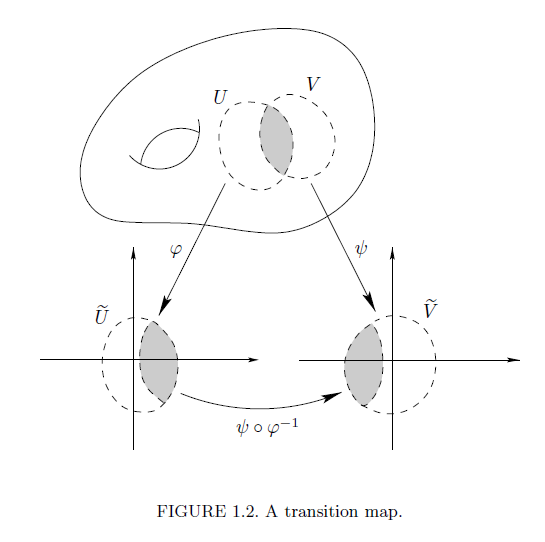什么样的相容条件，就决定了什么样的微分流形，比如要是 $\psi\circ\varphi^{-1}$ 及它的逆都是$C^k$的，那么我们可以类似的定义$C^k-$微分流形。在这里讨论的所有坐标卡相容，都是光滑相容。
两两相容的一族坐标卡 $\{U_\alpha,\varphi_\alpha\}$并且$\bigcup_{\alpha\in\lambda} U_\alpha=M$，就称为一个地图册，图册或者坐标卡集(atlas)，要是所有互相相容的坐标卡，都已经放在了同一个地图册里，称这样的地图册是极大地图册(maximal atlas)。
那么这样，我们就可以定义本篇最重要的概念了：
Definition 7.2.1 微分结构 (Differential structure)
一个极大地图册，就是定义在$n$-维拓扑流形上的微分结构。
我觉得入门微分流形的第一个重要的地方就是理解什么是微分结构。他定义前提是在拓扑流形上，包含了坐标卡，相容性条件（转移函数），极大地图册。
那么当然会有问题了，我要验证一个拓扑流形是微分流形，就需要给出微分结构，也就是极大地图册，而极大地图册中可以有很多坐标卡，可数个，不可数个都有可能，我不可能写的出来。好在有定理保证了，只要给出了一个地图册，也就是定义域覆盖住了$M$，且其中的坐标卡两两相容，那么这个地图册就唯一的包含在一个极大地图册中，从而使拓扑流形$M$成为微分流形：
Lemma 7.2.2
(1) 每一个地图册唯一的包含在一个极大地图册中；
(2) 两个地图册，都被包含在同一个极大地图册中当且仅当这两个地图册是相容的。
证明思路：
(1) 现在已经有一个地图册$\mathcal{A}$，我们这样给出一个新的集合：$\overline{\mathcal{A}}$是所有与$\mathcal{A}$相容的坐标卡的集合。
接着首先说明$\overline{\mathcal{A}}$就是一个地图册：其中任意给定的两个坐标卡，都和$\mathcal{A}$中的任意一个坐标卡相容，通过$\mathcal{A}$中的坐标卡作为桥梁，即可说明相容性。
极大性从构造中就可以看出。
唯一性是因为假设还有极大地图册，必然包含于$\overline{\mathcal{A}}$，反过来$\overline{\mathcal{A}}$也包含于极大地图册中，所以唯一。
(2) 充分性：这两个地图册相容，从而可以合并成一个新地图册，由(1)知他们包含在同一个极大地图册中。
必要性：极大地图册中坐标卡两两相容，从而原本的两个地图册相容。

有了这个定理保证，在验证微分结构的时候就方便了很多。
比方说我们就可以验证，之前在拓扑流形中举的几个例子$\mathbb{R}^n$，$S^n$，有限维线性空间，$GL(n,\mathbb{R})$，都可以给出相容的坐标卡，从而他们也是光滑流形。

综上，我们要证明一个空间 $M$ 是微分流形，首先要说明他是一个拓扑流形，这就意味着要先给出 $M$ 上的拓扑，验证其是一个拓扑流形之后再给出一个覆盖住 $M$ 的地图册，从而有了微分结构。
事实上，$M$上的拓扑是由地图册唯一决定的，我们可以把欧氏空间的拓扑局部的赋予到流形上，这样就避免了在 $M$ 上重新定义拓扑。

Theorem 7.2.3 从集合到光滑流形
给定集合 $M$ 的一个覆盖 $\{U_\alpha\}$，且在每个$U_\alpha$上有一个单射 $\varphi_\alpha: U_\alpha\rightarrow \mathbb{R}^n$，并且满足下列条件：
(1) $\forall \alpha$，$\varphi_\alpha(U_\alpha)$是$\mathbb{R}^n$中开集；
(2) $\forall \alpha,\beta$，$\varphi_\alpha(U_\alpha\cap U_\beta),\varphi_\beta(U_\alpha\cap U_\beta)$也都是$\mathbb{R}^n$中开集；
(3) 当$U_\alpha\cap U_\beta\neq\emptyset$时，$\varphi_\alpha\circ\varphi^{-1}_\beta:\varphi_\beta(U_\alpha\cap U_\beta)\rightarrow \varphi_\alpha(U_\alpha\cap U_\beta)$是光滑的；
(4) 存在可数多个 $U_\alpha$覆盖住 $M$；
(5) 对$M$上不同的两点$p,q$，他们要不包含在同一个$U_\alpha$中，要不分别包含在不相交的两个集合$U_\alpha,U_\beta$中。
这样$M$是一个光滑流形，并且有唯一的拓扑和微分结构$\{U_\alpha, \varphi_\alpha\}$.
证明思路：
这个定理的条件很多，但其实各司其职(1)(2)(3)让我们可以对$M$定义拓扑，并且(3)保证了坐标卡的相容性，从而有唯一微分结构，(4)保证第二可数性，(5)保证Hausdorff.
先在$M$上定义拓扑：
记$\tilde{U}_\alpha=\varphi_\alpha(U_\alpha)\subset\mathbb{R}^n$，从而$\varphi_\alpha:U_\alpha\rightarrow\tilde{U}_\alpha$是双射，取$\forall V\subset \tilde{U}_\alpha$是开集，定义$\varphi_\alpha^{-1}(V)$是$M$中开集。接着证明在(1)(2)(3)条件下，像$\varphi_\alpha^{-1}(V)$这样的集合构成了$M$的拓扑基，从而有了在$M$上的拓扑：
记$W$为$\tilde{U}_\beta$中开集，那么可以知道$\varphi_\alpha\circ\varphi_\beta^{-1}(W)$为$\tilde{U}_\alpha$中的开集，从而$\forall p\in\varphi_\alpha^{-1}(V)\cap\varphi_\beta^{-1}(W)$，$p\in\varphi^{-1}_\alpha(V\cap \varphi_\alpha\circ\varphi_\beta^{-1}(W))=\varphi_\alpha^{-1}(V)\cap\varphi_\beta^{-1}(W)$，这样就证明了把欧氏空间中的开集通过 $\varphi$ 逆回去确实成为了$M$上的拓扑基。
第二可数是因为可数个$U_\alpha$，每个同胚于欧氏空间，从而有可数拓扑基，逆回到$M$上，总共的拓扑基依旧是可数的；Hausdorff是显然的。
这个拓扑的唯一性：首先由于$\varphi$是单射和(1)就意味着$M$上的拓扑不能比我们定义的拓扑更细，否则映到欧式空间就不是开集了。其次要保证$\varphi$的连续性，$M$上的拓扑必然包含我们定义的拓扑，同时不能比我们定义的更粗了。

在Nigel Hitchin的讲义中类似通过坐标卡定义了$M$上的拓扑：条件和定理中的条件是一样的，定义$V\subset M$是开集，当且仅当$\forall \alpha, \varphi_\alpha(V\cap U_\alpha)$是$\mathbb{R}^n$中开集。这与我们定义的拓扑是等价的。
总而言之，流形的本质是他不像欧氏空间是平直的，流形是一个扭曲的空间，我们有的只是局部性质。
参考：
John M. Lee.  Introduction to Topological Manifolds. Graduate Texts in Mathematics, Vol. 218. Springer-Verlag, New York, 2000.
N. Hitchin.   DIFFERENTIABLE MANIFOLDS Course C3.1b 2012 .
厦门大学宋翀老师讲义.


展开全文• 各位这是清华周坚老师的微分流形讲义,对想考请华博士的人来说不无好处.大家也可以看看了解一下,个人认为不错. 文件可用scientific workplace打开.
•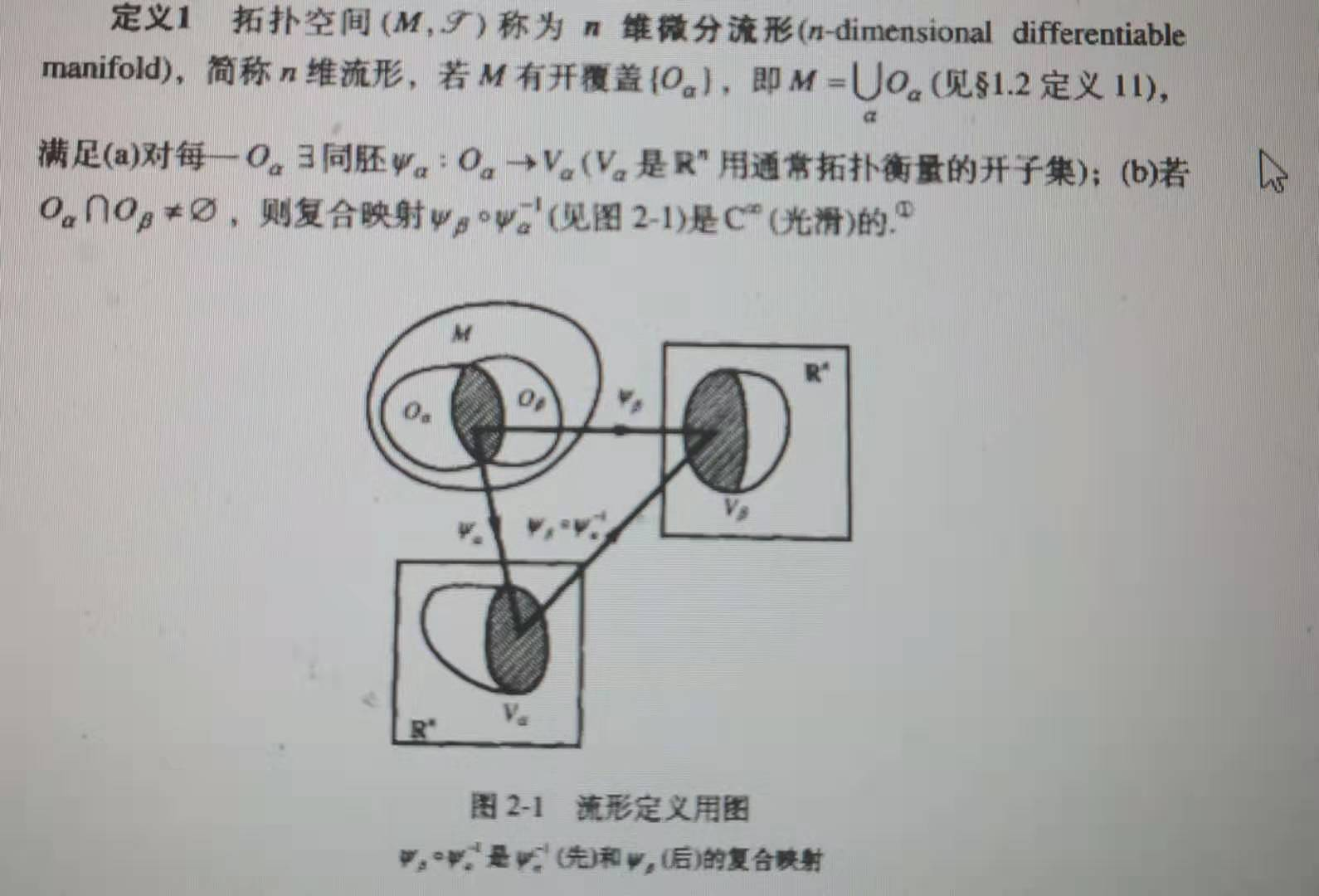展开全文• ## 微分流形简介

千次阅读 2019-03-20 17:18:19
1、欧氏空间流形 流形上每一点的邻域和欧氏空间的一个开集同胚，在此邻域可引入局部坐标系。流形可以看作一块块"欧氏空间"粘合起来。 coordinate patches 【与的一个开集同胚，则称为m维流形】 2、chart：（称为...
• 23.书名：微分流形与黎曼几何(英文版第2版修订版) 书号：978-7-115-16599-2 原书名：An Introduction to Differentiable Manifolds and Riemannian Geometry 分类：数学与统计 基础数学 几何 丛书名：图灵原版数学...math
• 由于种种原因要恶补一下微分流形和黎曼几何，吸取一下“前辈”们的经验，也希望大家能提供一些更好的经验！ 1 自几何佳缘 在这方面我是很有感受的。我整理了一些心得笔记，打算以后给学生上课的时候，把...
• 【2】《物理学家用微分几何》 【3】《微分几何入门与广义相对论》 一、连续性 在拓扑空间中因为没有度量的概念，因此用开集的逆像仍然是开集来定义连续性，如下举例: 备注：拓扑里的开集、闭集可以理解是实数轴开...
• 切向量，切空间设M为一m维微分流形， 为M上过点p的一条可微曲线， 为任意给定正数. 令 , ， 也即 , 表示 沿向量 的方向导数. 在 上定义相切关系: 由等价关系的自反性、对称性、传递性可知相切关系为一等价关系. 有了...可微偏导数一定存在
• 翻译自瓦内尔所著Foundations of Differentiable Manifolds and Lie Groups数学
• (Universitext) Serge Lang (auth.)-Introduction to Differential Manifolds-Springer-Verlag New York 入门经典 高清英文
• 这着实是本大部头，全书共计1900多页，涵盖了机器学习和深度学习相关的多个数学学科，包括线性代数，拓扑学、微分计算和最优化理论等。这本书的PDF电子版现已放出，需要的读者可以免费下载。 资源获取： 添加学习...
• 微分方程: 常微分方程定性理论的初步内容（不用涉及混沌） 偏微分方程的基本分类，理解分离变量法的原理，掌握积分变换法，了解格林函数法即可。 3.拓扑与几何 了解最基本的点集拓扑、流形论、向量分析的基本概念，...
• 由于种种原因要恶补一下微分流形和黎曼几何，吸取一下“前辈”们的经验，也希望大家能提供一些更好的经验！   1 自几何佳缘 在这方面我是很有感受的。我整理了一些心得笔记，打算以后给学生上课的时候，把这些...工具 扩展
• 熟知微分流形中子流形的定义。一个不难被提出的简单的问题是 问题对微分流形$M$，何种等价关系$\sim$可以使得商拓扑空间$M/\sim$具有微分流形的结构，使得自然映射$M\to M/\sim$是光滑的？ 这个问题在微分流形...
• 在数学中，特别是在微分几何和代数几何中，复流形是具有复结构的微分流形，即它能被一族坐标邻域所覆盖，其中每个坐标邻域能与n维复线性空间中的一个开集同胚，从而使坐标区域中的点具有复坐标 (z1,…,zn)，而对两个...数学基础
• 参考书籍：陈维桓《微分流形初步》，陈省身《微分几何》讲义 前置要求：代数结构，线性代数，张量代数，微分流形的初步了解 黎曼几何是现代几何学的重要概念，其理论已经深刻应用于广义相对论、机器学习。流形...抽象代数
• ## 黎曼流形

千次阅读 2010-12-05 11:24:00
黎曼流形是具有黎曼度量的微分流形，换句话说，这个流形上有一个对称 正定 协变 二阶张量场， 亦即每一点处有一个2阶正定矩阵。给了度量以后， 我们就可以向数学分析里做的那样，建立起微积分的理论。
• 1.什么是黎曼流形定义：黎曼流形（Riemannian manifold）是一个微分流形，其中每点p的切空间都定义了点积，而且其数值随p平滑地改变。它容许我们定义弧线长度、角度、面积、体积、曲率、函数梯度及向量域的散度。...manifold
• 文章目录概念与定义微分流形张量场与微分形式联络与曲率 概念与定义 微分流形 拓扑流形：　设MMM是一个Hausdorff拓扑空间，若对每一点p∈Mp\in Mp∈M，都有ppp的一个邻域UUU与Rm\mathrm{R}^mRm的一个开集同胚，则MMM...微分几何 基础数学
• 现代微分几何的研究对象是所谓的微分流形。估计有很多读者在很久以前就听说过这个词，但是一直不知道它的意思。为了引入微分流形，本文介绍必要的拓扑学知识。古典微积分的研究对象是函数和向量函数，构建它们的载体...
• Rimannian流形上二阶积分微分方程对粘弹性模型的可及性研究论文
• 上一篇定义了光滑微分流形，这一篇在其上定义光滑函数，并且定义流形之间的光滑映射。
• 我不知道大家学没学曲线论和曲面论，但我就当大家学了，我以后可能会把这种 微分几何的内容写一下。另外上期有同学说要讲相流，那么下节我就把相流放在单参群里讲一下吧。一、曲面上的测地线考虑在曲面 上的曲线 ，...
• 一、微分形式、楔形积(外积)定义1 ： 称为矢量空间 上的 形式，若 为全反称的，或写为 ，其中 为 上的全体 型张量.由此定义出可快速得出如下结论：对在任意基底下的分量，有 ；凡重复具体指标者必为0. ......